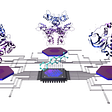# INTRODUCTION

In this article, I will explain and implement the well-known Traveling Salesman Problem aka TSP with a special focus on subtour elimination methods. We will use python to implement the MILP formulation. The dataset contains the coordinates of various cities of India. The aim is to find the shortest path that covers all cities. We will cover the following points in this article

1. Input the data and visualize the problem
2. Model TSP as ILP formulation w/o Subtour constraints
3. Implement Subtour Elimination Method 1: MTZ’s Approach
4. Implement Subtour Elimination Method 2: DFJ’s Approach
5. Compare MTZ’s formulation and DFJ’s formulation
6. Conclusion

# 1 Input the data and problem visualization

The CSV file “tsp_city_data.csv” contains the names of cities in India with their latitude and longitude information. The first city “Delhi” is assumed to be the starting point of the trip (depot). The data input to TSP model is the distance matrix which stores the distance (or travel time or cost) from each city (location) to every other city. Thus, for a traveling salesman problem for N cities (location), the distance matrix is of size N x N. The variable no_of_locs in the code is used to define the first n no. of cities we want to include in our TSP problem data. The value is set at 6 for now. The python pandas library is used to read CSV file and distance matrix “dis_mat”.

`#import libraries%matplotlib inlineimport pulpimport pandas as pdfrom scipy.spatial import distance_matrixfrom matplotlib import pyplot as pltimport timeimport copy`

The function “plot_fig” is used to plot the data and visualize the problem and the function “get_plan” takes the LP solution and returns all the subtours present in the solution.

`#This function takes locations as input and plot a scatter plotdef plot_fig(loc,heading="plot"):plt.figure(figsize=(10,10))    for i,row in loc.iterrows():        if i==0:            plt.scatter(row["x"],row["y"],c='r')            plt.text(row["x"]+0.2, row["y"]+0.2, 'DELHI (depot) ')        else:            plt.scatter(row["x"], row["y"], c='black')            plt.text(row["x"] + 0.2, row["y"] + 0.2,full_data.loc[i]['CITy'] )        plt.ylim(6,36)        plt.xlim(66,96)        plt.title(heading)# this function find all the subtour in the LP solution.def get_plan(r0):    r=copy.copy(r0)    route = []    while len(r) != 0:        plan = [r]        del (r)        l = 0        while len(plan) > l:            l = len(plan)            for i, j in enumerate(r):                if plan[-1] == j:                    plan.append(j)                    del (r[i])        route.append(plan)    return(route)`

The following code below reads data from the CSV file and creates a distance matrix for TSP problem.

`# set no of citiesno_of_locs=6data=pd.read_csv("tsp_city_data.csv")full_data=data.iloc[0:no_of_locs,:]d=full_data[['x','y']]dis_mat=pd.DataFrame(distance_matrix(d.values,d.values),\                       index=d.index,columns=d.index)print("----------data--------------")print(full_data)print("-----------------------------")plot_fig(d,heading="Problem Visualization")   plt.show()`

# 2 Model TSP in ILP without Subtour elimination constraints

TSP problem can be modeled as Integer Linear Program. The LP model is explained as follows

Data

N= Number of location including depot (starting point)
Ci,j = Edge cost from node i to node j where i,j= [1…N]

Decision Variable

xi,j = 1 if solution has direct path from node i to j, otherwise 0

The LP model is formulated as follows

The objective (1) minimize the cost of the tour. Constraints (2) and (3) ensures that for each node, there is only one outflow and inflow edge respectively. Thus, each node is visited only once. Constraint (3) restrict outflow to one’s own node.

In this article, python’s PuLP library is used for implementing MILP model in python. PuLP is an LP modeler written in Python. PuLP can call a variety of LP solvers like CBC, CPLEX, GUROBI, SCIP to solve linear problems. It can be installed from the link “https://pypi.org/project/PuLP/”. The CBC solver is preinstalled in the PuLP library while one has to install other solvers like GUROBI, CPLEX separately to use in PuLP. In this implementation, CBC is used as LP solver.

`model=pulp.LpProblem('tsp',pulp.LpMinimize)#define variablex=pulp.LpVariable.dicts("x",((i,j) for i in range(no_of_locs) \                             for j in range(no_of_locs)),\                            cat='Binary')#set objectivemodel+=pulp.lpSum(dis_mat[i][j]* x[i,j] for i in range(no_of_locs) \                      for j in range(no_of_locs))# st constraintsfor i in range(no_of_locs):    model+=x[i,i]==0    model+=pulp.lpSum(x[i,j] for j in range(no_of_locs))==1    model += pulp.lpSum(x[j, i] for j in range(no_of_locs)) == 1status=model.solve()#status=model.solver()print("-----------------")print(status,pulp.LpStatus[status],pulp.value(model.objective))route=[(i,j) for i in range(no_of_locs) \           for j in range(no_of_locs) if pulp.value(x[i,j])==1]print(get_plan(route))`
`plot_fig(d,heading="solution Visualization")arrowprops = dict(arrowstyle='->', connectionstyle='arc3', edgecolor='blue')for i, j in route:    plt.annotate('', xy=[d.iloc[j]['x'], d.iloc[j]['y']],\                      xytext=[d.iloc[i]['x'], d.iloc[i]['y']],\                     arrowprops=arrowprops)`

The optimal solution given by the LP model has subtours i.e

1. Tour 1 : Delhi > Nagpur > Rajkot
2. Tour 2 : Kolkata > Dispur > Agartala

The solution given by the model has 2 tours but what required is the single tour that starts with the depot (Delhi) and visits all locations one by one and ends at Delhi. To solve this problem and to get the desired single tour, the subtour elimination constraints need to be added in the LP Model.

There are 2 well-known formulations DSF and MTZ (named after their authors). This article covers both the ideas and the implementation in python.

# 3. MTZ Method for subtour elimination

This formulation was proposed by Miller, Tucker, Zemlin. To eliminate subtours, continuous decision variables representing times at which a location is visited is added. Variable for all locations except depot node is added. ti= time at which location i is visited, i =[2,…N] Finally what is required are the constraint

# How does constraint (5) remove subtours ?

Lets takes an previous example and take the subtour Kolkata (k) > Dispur(d) > Agartala(a)

So adding constraint (5) will eliminate the subtour.

The complete Lp model is formulated as follows

Data

N= Number of location including depot (starting point)
Ci,j = Edge cost from node i to node j where i,j= [1…N]

Decision Variable

xi,j = 1 if solution has direct path from node i to j, otherwise 0
ti = time at which location i is visited , i =[2,…N]

The LP model is formulated as follows

The MTZ’s formulation is implemented in python as shown below.

`start_t=time.time()model=pulp.LpProblem('tsp',pulp.LpMinimize)#define variablex=pulp.LpVariable.dicts("x",((i,j) for i in range(no_of_locs) \                                for j in range(no_of_locs)),\                           cat='Binary')t = pulp.LpVariable.dicts("t", (i for i in range(no_of_locs)), \                             lowBound=1,upBound= no_of_locs, cat='Continuous')#set objectivemodel+=pulp.lpSum(dis_mat[i][j]* x[i,j] for i in range(no_of_locs) \                      for j in range(no_of_locs))# st constraintsfor i in range(no_of_locs):    model+=x[i,i]==0    model+=pulp.lpSum(x[i,j] for j in range(no_of_locs))==1    model += pulp.lpSum(x[j, i] for j in range(no_of_locs)) == 1#eliminate subtourfor i in range(no_of_locs):    for j in range(no_of_locs):        if i!=j and (i!=0 and j!=0):            model+=t[j]>=t[i]+1 - (2*no_of_locs)*(1-x[i,j])status=model.solve()#status=model.solver()print("-----------------")print(status,pulp.LpStatus[status],pulp.value(model.objective))route=[(i,j) for i in range(no_of_locs) \           for j in range(no_of_locs) if pulp.value(x[i,j])==1]print(route)plot_fig(d,heading="solution Visualization")arrowprops = dict(arrowstyle='->', connectionstyle='arc3', edgecolor='blue')for i, j in route:    plt.annotate('', xy=[d.iloc[j]['x'], d.iloc[j]['y']],\                      xytext=[d.iloc[i]['x'], d.iloc[i]['y']],\                     arrowprops=arrowprops)print("time taken by MTZ formulation = ", time.time()-start_t)`

# 4. DFJ Method for subtour elimination

This formulation was proposed by Dantzig, Fulkerson, Jhonson. To eliminate subtours, for every set S of cities, add a constraint saying that the tour leaves S at least once.

# How does this constraint eliminate subtours?

Let's take the same example and take a set Si= {kolkata, Dispur, Agartala} and the rest of the cities be represented by s′i={ Delhi(del), Rajkot(r), Nagpur(n)}
Now as per constraint (15), the new constraint added is as follows

since there is no edge going to any other node in this set (due to subtour), this equation is not satisfied for the set Si= {{kolkata, Dispur, Agartala}. So, by adding constraint (15), this solution becomes infeasible and all subtours will be eliminated.

# Modification in DFJ Method

For N cities, the number of possible sets adds up to 2^n i.e the number of constraints grows exponentially. So, instead of adding constraints for all the possible sets, only some constraints are added. Given a solution to LP model(without having subtour elimination constraints) with subtours, one can quickly find the subset for which DFJ subtour constraint is eliminated. In the example above, one needs to add only 2 constraints and not 2^5 constraints.

So, the higher-level algorithm is as follows

# Higher-level Algorithm for DFJ

step 1. Solve TSP problem with LP formulation w/o Subtour Constraints

step 2. If no subtour present in the current solution, goto step 6

step 3. Add subtour constraint only for the subtours present in the current solution.

step 4. Solve TSP problem with newly added constraint.

step 5. goto step 2

step 6. Return the final TSP solution

The above-mentioned algorithm is implemented as follows

`start_t_1=time.time()model=pulp.LpProblem('tsp',pulp.LpMinimize)#define variablex=pulp.LpVariable.dicts("x",((i,j) for i in range(no_of_locs) \                                for j in range(no_of_locs)),\                           cat='Binary')#set objectivemodel+=pulp.lpSum(dis_mat[i][j]* x[i,j] for i in range(no_of_locs) \                      for j in range(no_of_locs))# st constraintsfor i in range(no_of_locs):    model+=x[i,i]==0    model+=pulp.lpSum(x[i,j] for j in range(no_of_locs))==1    model += pulp.lpSum(x[j, i] for j in range(no_of_locs)) == 1    status=model.solve()route=[(i,j) for i in range(no_of_locs) \           for j in range(no_of_locs) if pulp.value(x[i,j])==1]route_plan=get_plan(route)subtour=[]while len(route_plan)!=1:    for i in range(len(route_plan)):            #print(route_plan[i])model+=pulp.lpSum(x[route_plan[i][j],route_plan[i][j]]\                              for j in range(len(route_plan[i])))<=\                              len(route_plan[i])-1    status=model.solve()    route = [(i, j) for i in range(no_of_locs) \                 for j in range(no_of_locs) if pulp.value(x[i, j]) == 1]route_plan = get_plan(route)        subtour.append(len(route_plan))print("-----------------")print(status,pulp.LpStatus[status],pulp.value(model.objective))print(route_plan)print("no. of times LP model is solved = ",len(subtour))print("subtour log (no. of subtours in each solution))",subtour)print("Time taken by DFJ formulation = ", time.time()-start_t_1)plot_fig(d,heading="solution Visualization")arrowprops = dict(arrowstyle='->', connectionstyle='arc3', edgecolor='blue')for i, j in route_plan:    plt.annotate('', xy=[d.iloc[j]['x'], d.iloc[j]['y']],\                     xytext=[d.iloc[i]['x'], d.iloc[i]['y']],                     arrowprops=arrowprops)plt.show()#print("total time = ",time.time()-start)`

# Compare MTZ’s Formulation vs DFJ’s formulation

Since two approaches for subtour elimination have been discussed in this article, it's time to compare the two. MTZ’s approach introduces n² constraints (one for each pair (i,j) where i, j=[1..n]) while DFJ’s approach introduces subtour constraints for all possible sets of locations i.e 2^n for n locations. Thus, MTZ’s approach adds a polynomial number of constraints while DFJ’s approach introduces an exponential number of constraints.

In terms of decision variables, MTZ approach introduces n new decision variables (titi for i =[1..n]).ON the other hand, DFJ introduces no new decision variable. MTZ’s approach has to be solved only once to get an optimal solution While DFJ is generally implemented as a modified version and it is solved iteratively ( i.e LP model has to be solved multiple times with new subtour constraints added every time).

There is no clear winner among the two. For some problems, DFJ gives solutions faster than MTZ and for some problems, MTZ is faster. But DFJ has an efficient branch and bound approach due to which it becomes more efficient than MTZ. Also, MTZ’s formulation is weaker i.e the feasible region has the same integer points but includes more fractional points.

# Conclusion

In this article, MILP formulation of TSP is explained with a special focus on subtour elimination approaches. TSP problem is a special case of Vehicle Routing Problem (VRP) with no. of vehicle equal to 1. But, subtour elimination is a core issue in VRP as well which is solved by using the same techniques. In this article, the TSP problem is solved for only 6 cities to simplify the explanation of subtour elimination. The CSV file uploaded in the Github repository contains data of 27 cities. One can try to solve the problem for more number of cities.

--

--

--

## More from The Startup

Get smarter at building your thing. Follow to join The Startup’s +8 million monthly readers & +756K followers.

## Richard Stallman and Linux## Nuke AWS Resources## A Git hack that you might need being a coder.## How to Back Up MySQL Database on Alibaba Cloud ECS Ubuntu 16.04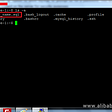## How to enable a serverless first cloud centre of excellence## the simulator problem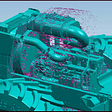## GitHub Actions + ACK: A Powerful Combination for Cloud-Native DevOps Implementation## What is new in Nx 13.10?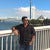## Aayush Aggarwal

AI Reseacher | IIT Bombay Alumnus

## SNOMED CT: An Introduction — Part 2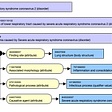## AI & ML in Food Industry## Do we need BIM Manager Positions?## Undruggable targets: the major challenge for modern drug discovery